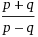# Logarithm formula help

Michael_Light

## Homework Statement

If log4 N=p and log12 N=q, show that

log3 48=## The Attempt at a Solution

I tried by substituting p and q intobut i couldn't get the required answer. Can anyone help?

## Answers and Replies

Homework Helper

Use the formula $$\log_ab=\frac{\log_cb}{\log_ca}$$

Homework Helper

## Homework Statement

If log4 N=p and log12 N=q, show that

log3 48= View attachment 32226

## The Attempt at a Solution

I tried by substituting p and q into View attachment 32226 but i couldn't get the required answer. Can anyone help?

Well, can you post your work, so that we can help you continue it? You are on the right track. Some of the logarithmic identities you should remember is (a, x, y are all positive real number):

• loga(xy) = logax + logay
• $$\log_{a} \left( \frac{x}{y} \right) = \log_{a}(x) - \log_{a}(y)$$
• $$\log_{a} x = \frac{1}{\log_{x} a}$$
• $$\log_{a} b \times \log_{b} c = \log_{a} c$$ or $$\log_{a} b =\frac{\log_{c} b}{\log_{c} a}$$

There's another way.

Note that, we have: log4 N = p, and log12 N = q.

And we want to calculate: log348. If you look at that closely, you'll discover that: 48 = 4.12

So log348 = log3(4.12)

By playing with some of the identities I gave you above, you'll soon arrive at the desired answer. :)

Michael_Light

Thanks! I finally managed to solve the question by proving that (p+q)/(p-q) is equal to L.H.S.

Just to ask is it possible for me to obtain (p+q)/(p-q) from what i have done below? How should i continue?

log348
= log3 (4.12)
= log34 + log312
= (log3 N)/p + (log3N)/q
= (q log3N + p log3N)/pq
=[(p+q)log3N]/pq

Is it possible to continue?

Homework Helper

Thanks! I finally managed to solve the question by proving that (p+q)/(p-q) is equal to L.H.S.

Just to ask is it possible for me to obtain (p+q)/(p-q) from what i have done below? How should i continue?

log348
= log3 (4.12)
= log34 + log312
= (log3 N)/p + (log3N)/q
= (q log3N + p log3N)/pq
=[(p+q)log3N]/pq

Is it possible to continue?

So, you are stuck in expressing log3N in terms of p = log4N, and q = log12N, right? First, you should notice that 3 = 12/4. So, we have:

$$\log_{3} N = \frac{1}{\log_{N} 3} = \frac{1}{\log_{N} \left( \frac{12}{4} \right)} = ...$$

Can you finish it from here? :)

Michael_Light

So, you are stuck in expressing log3N in terms of p = log4N, and q = log12N, right? First, you should notice that 3 = 12/4. So, we have:

$$\log_{3} N = \frac{1}{\log_{N} 3} = \frac{1}{\log_{N} \left( \frac{12}{4} \right)} = ...$$

Can you finish it from here? :)

Thousands of thanks VietDao29! I solved it. Thanks again. ^^

Staff Emeritus
$$\log_{4}\,N=p\quad\Leftrightarrow\quad4^{p}=N\quad\text{ and }\quad\log_{12}\,N=q\quad\Leftrightarrow\quad12^{q}=N$$